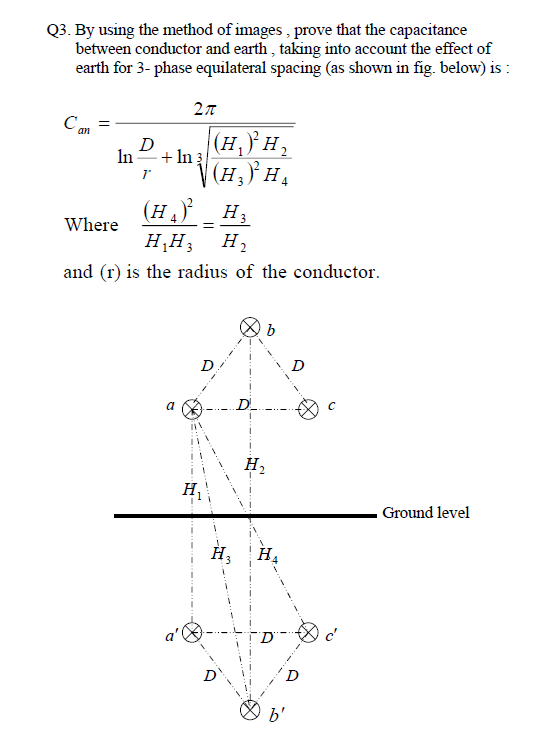# Q3. By using the method of images, prove that the capacitance between conductor and earth, taking into account the effect of earth for 3- phase equilateral spacing (as shown in fig. below) is 2π Where and (r) is the radius of the conductor Ground level

Questionhelp_outlineImage TranscriptioncloseQ3. By using the method of images, prove that the capacitance between conductor and earth, taking into account the effect of earth for 3- phase equilateral spacing (as shown in fig. below) is 2π Where and (r) is the radius of the conductor Ground level fullscreen

### Want to see the step-by-step answer?